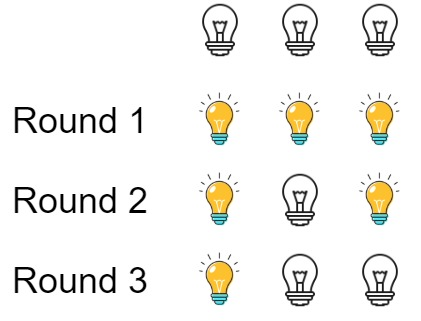# Leetcode 319 - Bulb switcher

Note:

• If a bulb wants to be turned on in the end, it has to been switched for odd times.
• For Kth bulb, if there is a factor between [1, k], say m, we can be sure k/m is also in between [1,k].
• So if k has odd number of factors, it must be on in the end.
• To let k have odd number of factors, it must be a Square number.
• So the real question is how many square numbers in [1,n]?
• Based on some math deduction, it’s floor(sqrt(n)).

Question:

There are n bulbs that are initially off. You first turn on all the bulbs, then you turn off every second bulb.

On the third round, you toggle every third bulb (turning on if it’s off or turning off if it’s on). For the ith round, you toggle every i bulb. For the nth round, you only toggle the last bulb.

Return the number of bulbs that are on after n rounds.

Example:Code: